# Supervised Machine Learning and Classification

​​​​​​​​​​​​​​​​​​​​​​​​​​Supervised learning is a predictive modelling technique, used in machine learning. It is characterized by the training of a model on a labeled or “training” dataset, which includes a set of input variables and their corresponding output. This allows the model to establish rules between the input and output data, in order to map new unlabelled inputs to their unknown output.

​​​​​​​​​​​​​​​​​​​​​​​​Supervised machine learning can be roughly separated into two branches: regression and classification. In a previous article, we touched on supervised machine learning and dove into the regression models including simple and multiple linear regression, Poisson regression and Support Vector Regression (SVR).

Now, we will look at the classification method as well the classification evaluation metrics and measures, used to evaluate a classification model. If you wish to learn more about the different classification algorithms and their use at Linedata, please look out for upcoming articles.

### Classification​​​​​​​In a previous article, we had focused on regression models, which predict a continuous-valued output based on an independent input variable. On the other hand, in classification models, the output variable is discrete. This implies that the model is trained in such a way to render a discrete output (y) for some input data (x). The output is therefore a labelling or a class, thus reflecting the term “classification”.

For instance, if we are trying to predict the price of a car, using a classification model will allow us to classify that particular car within a predetermined range of prices, while a regression model would attempt at predicting the exact price.

In the case in which only two classes can be outputted by the model, we talk about Binary Classification. On the other hand, when more than two output classes are possible, we refer to Multi-class classification.

Classification models are most widely used for image classification, document classification, NLP, or even for fraud detection.

### Classification evaluation metrics

Once a model has been determined and implemented, it is important to establish how effective the model is. To do so, different evaluation metrics can be used and chosen carefully, as the choice of the metrics will influence how the performance of the model is measured and compared.

One of the most popular and easiest ways to measure the performance of a classification model is the confusion matrix. It is a tabular summary of the number of correct and incorrect predictions made by the model. It includes two dimensions: “Actual” and “Predicted” and each of these two dimensions has a “True Positive (TP)”, a “True Negative (TN)”, a “False Positive (FP)” and a “False Negative (FN)” cell.

The description of each term associated with this matrix is as follow :

• True Positive (TP) is used when the actual class and the predicted class are both Positive
• True Negative (TN) is used when the actual class and the predicted class are both Negative
• False Positive (FP) is used when the actual class is negative but the predicted class is Positive. This is also known as a Type 1 error.
• False Negative (FN) is used when the actual class is Positive but the predicted class is Negative This is also known as a Type 2 error.

In the case of a binary classification, the confusion matrix would be a 2 x 2 matrix with 4 values, as shown below: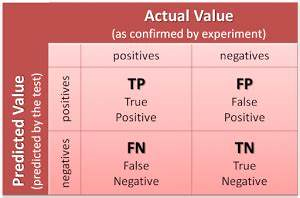###### Confusion matrix for a binary classification

In this case, the output variable can take two values : Positive or Negative. The columns represent the actual class of the input variables, while the rows correspond to the predicted class made by the model.

### Classification measures

Once the confusion matrix has been established, it can be used for further measures, which will help to achieve a better evaluation of the performance of the model. Some classification measures include: accuracy, precision, recall or sensitivity, specificity as well as F1-score.

a. Accuracy

Accuracy corresponds to the number of correct predictions made by the model. It represents the ratio between the number of correct predictions and the number of total predictions. It can be calculated using the values from the confusion matrix and the following formula: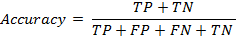​​​​​​​

We use accuracy as a metrics when True Positive and True Negative are more important.

b. Precision

Precision corresponds to the number of correct elements returned by the model. In other words, it is the ratio between the number of correctly classified positive classes and the total number of predicted positive classes. It can be calculated using the following formula: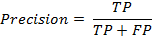We use the precision metrics whenever False Positive is most important.

c. Recall or Sensitivity

Recall, also known as Sensitivity, is defined as the number of positive classes returned by the model. It corresponds to the ratio between the number of correctly predicted positive classes and the total number of positive classes predicted. It can be calculated using the following formula: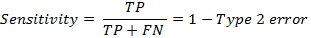We can use the sensitivity metrics whenever False Negative is the most important.

d. Specificity

Specificity corresponds to the number of negative classes predicted by the model. It can be determined by the ratio between the number of correctly predicted negative classes and the total number of negative classes predicted. It can be calculated using the following formula: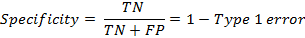​​​​​​​

e. F1-score

F1-score corresponds to a combination of the Sensitivity and the Precision metrics. It is used when there is no clear distinction between the two or when False Negative and False Positive are most important: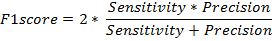### What’s next

Hence, we have explored the various measures that can be used to evaluate the performance of a classification model. For each application, the end user must choose which metrics to use, based on his/her classification problem. Now that we have understood this evaluation process, we will explore the main classification algorithms in the following two articles.